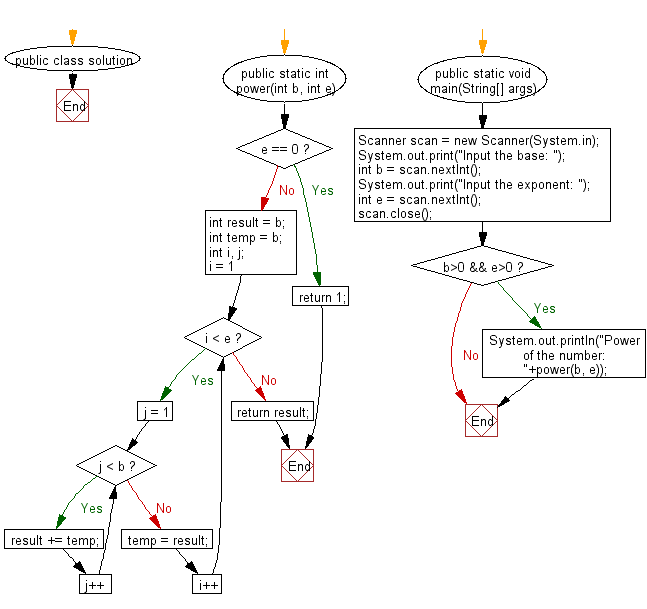﻿ Java Math Exercises: Calculate power of a number without using multiplication and division operators - w3resource# Java Math Exercises: Calculate power of a number without using multiplication and division operators

## Java Math Exercises: Exercise-16 with Solution

Write a Java program to calculate power of a number without using multiplication(*) and division(/) operators.

Sample Solution:

Java Code:

``````import java.util.*;
public class solution {
public static int power(int b, int e)
{
if (e == 0)
return 1;

int result = b;
int temp = b;
int i, j;

for (i = 1; i < e; i++) {
for (j = 1; j < b; j++) {
result += temp;
}
temp = result;
}

return result;
}

public static void main(String[] args) {
Scanner scan = new Scanner(System.in);
System.out.print("Input the base: ");
int b = scan.nextInt();
System.out.print("Input the exponent: ");
int e = scan.nextInt();
scan.close();
if (b>0 && e>0)
System.out.println("Power of the number: "+power(b, e));
}
}
``````

Sample Output:

``` Input the base:  2
Input the exponent:  5
Power of the number: 32
```

Flowchart:Java Code Editor:

What is the difficulty level of this exercise?

﻿

## Java: Tips of the Day

Parsing dates:

```import java.io.*;
import java.util.*;
import java.text.*;

String s = "2001/09/23 14:39";

SimpleDateFormat formatter = new SimpleDateFormat ("yyyy/MM/dd H:mm");
Date d = formatter.parse(s, new ParsePosition(0));
```